# Quadratic Inequalities in GMAT: Algebraic Method

Quadratic Inequalities is an important and often misunderstood topic in GMAT. But before we start, let us recall what a quadratic equation is and how is it represented.

The standard form of a quadratic equation is: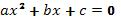And the roots of this standard equation are: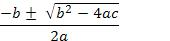where b2 - 4ac  is known as a Discriminant, ?.

This standard quadratic equation becomes an inequality if it is represented as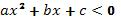(or > 0)

The first step in solving a quadratic inequality problem is to convert the equation into the standard equation format (if it is not given in the standard form) making zero on the right hand side.

The objective of solving any inequality for ‘x’ is to look for those values of ‘x’ which will make the inequality true.  These are known as the solutions to inequality and are usually represented in terms of intervals. While solving the quadratic equation, the roots are denoted as ‘x1’ and ‘x2’ which are real when the equation f(x) = 0.

The notation for an open interval is (x1, x2) and for closed intervals is [x1, x2]. The closed intervals include the end-points in the solutions while the open intervals don’t. Similarly, half closed intervals mean (-?, x1] and [x2, + ?).

Let’s look at a sample problem: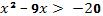Step 1: this equation is not given in the standard form, so let’s convert the right hand side so that the equation will be in the standard form.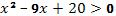Step 2: Factoring the equation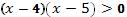Step 3: Find the intervals which satisfy the given condition.

Thus we see that x < 4 and x > 5 are possible solutions.

The method we have just discussed is commonly known as the ‘algebraic method’. Other methods used for solving quadratic inequalities are – the number line method and graphical method.

The number line method involves drawing the boundaries on the line and creating the intervals for investigation. Then pick a number from each interval and test it for the original inequality.  If the result happens to be true then that interval is a solution to the inequality.

The two real roots x1 and x2, as obtained from Step 3 of the algebraic approach as discussed in the above example, are plotted on the number line. Then the number line is divided into one segment and 2 rays.

Remember to keep the origin 0 as the test point.

Next substitute x = 0 in the quadratic inequality equation (converted into the standard form). In case the inequality is true then existence of the origin lay on the true segment. Hypothetically, if one segment is found to be a part of the solutions, then other segment would automatically be a part of the solutions owing to symmetric property of the parabolic curve.

The graphical method
involves drawing curves. Since the equations can also be represented as graphs, then depending on the type of inequality we have to choose the corresponding area. For an < 0 type of inequality the area lying below the x-axis is to be considered and vice-versa.

Thus, when the parabolic curve for f(x) lies above the x-axis, then the inequality is positive. The opposite is also true. Aspirants should note that there is no need to draw the parabola accurately but the rough sketch should be good enough to give an idea of two roots.

But care must be taken to accurately set the bent of parabola, i.e., upward or downward. There is one particular advantage of this method. It can be used to solve two or three quadratic inequalities by graphing two or three parabolas on the same coordinates.

Finally let us discuss some quick tips:

1. Time is always the biggest enemy in the exams so it’s most essential that problems should be solved as fast as possible. Moreover, applicants must also understand that many times a problem can be solved quickly by applying the method most suitable to the problem at hand. Some problems require an algebraic solution, while some are easier to solve by graphs. So applying the right method is very essential.

2. For multiple choice questions, it has been observed that substituting the most probable answers into the quadratic equation, or applying the algebraic method lead to the fastest results. The drawing of a graph or the number-line methods require more time.

Author :GoGMAT, founded in 2009, is an adaptive GMAT preparation platform developed by the best instructors in the industry (with 740+ GMAT scores and strong teaching experience). GoGMAT team consists of high profile members, successful entrepreneurs, experts in educational field, top-notch business school alumni with successful track records as investment bankers and management consultants. Our GMAT experts, university professors and successful entrepreneurs, analyzed GMAT test algorithms for almost 12 months.  We used their knowledge and skills as the basis to create one of the most efficient solutions in the world for GMAT preparation.

Disclaimer: The articles provided by GoGMAT are for informational purposes only and cannot be copied or redistributed without the written permission by GoGMAT. F1GMAT publishes guest posts by authors from all over the world. We do not accept any responsibility or liability for the information contained in the article or claims of copyright violation. However, we take immediate action when any copyright violations are reported. Report copyright violation1. Complete GMAT RC Questions in less than 1 minute and 50 seconds
3. Take Notes Effectively
4. Collect and Interpret Facts
5. Speed up Summary Creation
6. Remember Information
7. Question the Author

12. Learn to Answer GMAT organization of passage Question
13. Learn to identify the style/tone or attitude of the author

Mastering GMAT Critical ReasoningAfter you read F1GMAT’s Mastering GMAT Critical Reasoning Guide, you will learn:

How to overcome flawed thinking in GMAT Critical Reasoning?

How to spot Inconsistencies in Arguments

How to eliminate out of scope answer choices using Necessary and Sufficient Conditions

How to Paraphrase GMAT Critical Reasoning Question

How to Answer Assumption Question Type

How to Answer Conclusion Question Type

How to Answer Inference Question Type

How to Answer Strengthen Question Type

How to Answer Weaken Question Type

How to Answer bold-faced and Summary Question Types

How to Answer Parallel Reasoning Questions

How to Answer the Fill in the Blanks Question

Get F1GMAT's Newsletters (Best in the Industry)

• Ranking Analysis
• Post-MBA Salary Trends
• Post-MBA Job Function & Industry Analysis
• Post-MBA City Review
• MBA Application Essay Tips
• School Specific Essay Tips
• GMAT Preparation Tips